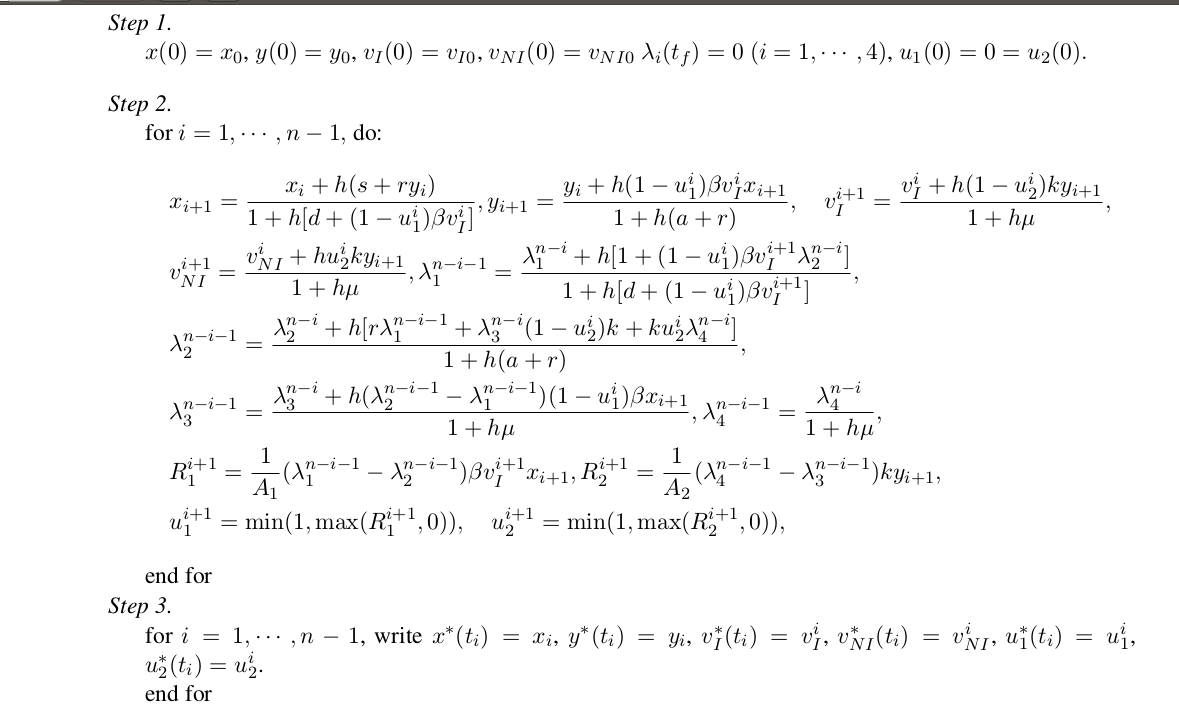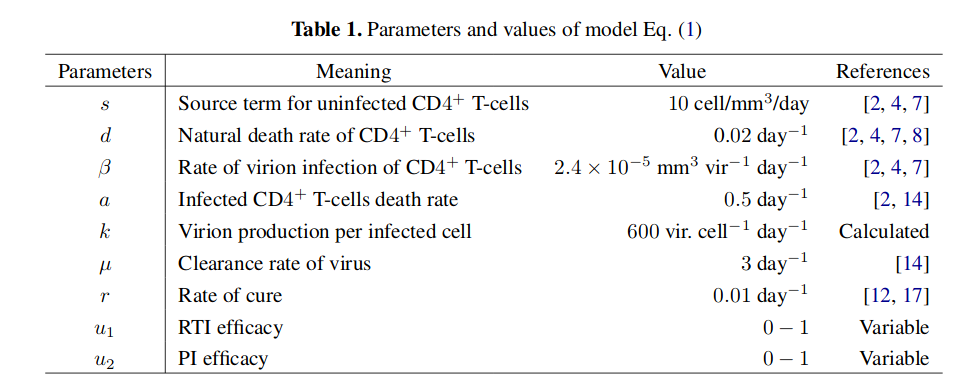Bottom Page

• 0 Vote(s) - 0 Average
• 1
• 2
• 3
• 4
• 5
 How can I program this algorithm with PythondocmathsProgrammer named TimPosts: 5 Threads: 1 Joined: Jul 2019 Reputation: 0 Likes received: 0 #1 Jul-08-2019, 09:20 AM (This post was last modified: Jul-08-2019, 09:27 AM by docmaths. Edited 2 times in total.) How can I program this algorithm with Python.Thank you very much. Additional Information :A1=2500; A2=250.ichabod801Bunny RabbitPosts: 4,231 Threads: 94 Joined: Sep 2016 Reputation: 272 Likes received: 1264 #2 Jul-08-2019, 10:50 AM What have you tried? We're not big on writing code for people here, but we would be happy to help you fix your code when you run into problems. When you do run into problems, please post your code in Python tags, and clearly explain the problem you are having, including the full text of any errors. That said, this is just a couple for loops and some basic math. x sub i just becomes x[i]. So, x[i + 1] = x[i] + h * (s + r * y[i]) / (...) ... Craig "Ichabod" O'Brien - xenomind.com I wish you happiness. Recommended Tutorials: BBCode, functions, classes, text adventuresscidamPosts: 681 Threads: 1 Joined: Mar 2018 Reputation: 91 Likes received: 103 #3 Jul-08-2019, 11:15 AM (This post was last modified: Jul-08-2019, 11:15 AM by scidam. Edited 1 time in total.) I don't think the problem is too hard. As far as I understand, t_f (t and lower index f, italic) in your formula stands for `t_i` when `i = n-1`; Even down-counting relations, which include lambda with indices (eq #5-#8), are consequently solvable. However, you need to have a lot of punctuality to implement this recurrent relation; intuitive variable naming is very important here. I just wrote some sketch here, you need to continue: ```defaults = {'s': 10, 'd': 0.02, 'beta': 2.4e-5, # define other values here 'h': 0.01 # h is time-step? you need to define it here. are we planning to use variable-step method? } initial_conditions = {'x0': 0, # other conditions } def equation(x, y, vi, vni, **defaults) i = 0 X, Y = [], [] xi = initial_conditions['x0'] yi = initial_conditions['y0'] while i < n: xi_1 = (xi + h*(s+r*yi))/(1 + h* ...) # complete the formula # write other formulae here # reassign values xi = xi_1 yi = yi_1 # save values X.append(xi_1) Y.append(yi_1) i += 1```This is likely some immunology model, may be modeling HIV infection dynamics.docmathsProgrammer named TimPosts: 5 Threads: 1 Joined: Jul 2019 Reputation: 0 Likes received: 0 #4 Jul-08-2019, 07:44 PM (This post was last modified: Jul-09-2019, 12:44 AM by scidam. Edited 2 times in total. Edit Reason: error tag added ) I wrote this program but apparently there is a problem. Can you help me? ```import numpy as np n=2501 lambd1=np.arange(n) lambd2=np.arange(n) lambd3=np.arange(n) lambd4=np.arange(n) x=np.arange(n) y=np.arange(n) vi=np.arange(n) vni=np.arange(n) u1=np.arange(n) u2=np.arange(n) x=200 y=80 vi=5000 vni=0 lambd1[n-1]=0 lambd2[n-1]=0 lambd3[n-1]=0 lambd4[n-1]=0 u1=0 u2=0 s=10 d=0.02 beta=2.4*10**-5 a=0.5 k=600 mu=3 r=0.01 h=100/(n-1) for i in range(n-1): x[i+1]=(x[i]+h*(s+r*y[i]))/(1+h*(d+(1-u1[i])*beta*vi[i])) print(x[i+1]) y[i+1]=(y[i]+h*(1-u1[i])*beta*vi[i]*x[i+1])/(1+h*(a+r)) vi[i+1]=(vi[i]+h*(1-u2[i])*k*y[i+1])/(1+h*mu) vni[i+1]=(vni[i]+h*hu2[i]*k*y[i+1])/(1+h*mu) lambd1[n-i-1]=(lambd1[n-i]+h*(1+(1-u1[i])*beta*vi[i+1]*lambd2[n-i]))/(1+h*(d+(1-u1[i])*beta*vi[i])) lambd2[n-i-1]=(lambd2[n-i]+h*(r*lambd1[n-i-1]+lambd[n-i]*(1-u2[i])*k+k*u2[i]*lambd4[n-i])/(1+h*(a+r)) lambd3[n-i-1]=(lambd3[n-i]+(lambd2[n-i-1]-lambd1[n-i-1])*(1-u1[i])*beta*x[i+1]*h)/(1+h*mu) lambd4[n-i-1]=lambd4[n-i]/(1+h*mu) R1[i+1]=((lambd1[n-i-1]-lambd2[n-i-1])*beta*vi[i+1])/A1 R2[i+1]=((lambd4[n-i-1]-lambd3[n-i-1])*k*y[i+1])/A2 u1[i+1]=min(1,max(R1[i+1],0)) u2[i+1]=min(1,max(R2[i+1],0))```Returned message : ``````Error: lambd3[n-i-1]=(lambd3[n-i]+(lambd2[n-i-1]-lambd1[n-i-1])*(1-u1[i])*beta*x[i+1]*h)/(1+h*mu) ^ SyntaxError: invalid syntax ``````ichabod801Bunny RabbitPosts: 4,231 Threads: 94 Joined: Sep 2016 Reputation: 272 Likes received: 1264 #5 Jul-08-2019, 08:09 PM You are missing a close parenthesis (')') at the end of line 38. It looks like you have one missing at the end of line 39 as well. When you have a syntax error, always check the line before if the line in the error seems fine. And in the future please post the full text of the error message. That would have saved me trying to find which line the error was for. Craig "Ichabod" O'Brien - xenomind.com I wish you happiness. Recommended Tutorials: BBCode, functions, classes, text adventuresdocmathsProgrammer named TimPosts: 5 Threads: 1 Joined: Jul 2019 Reputation: 0 Likes received: 0 #6 Jul-08-2019, 08:14 PM (This post was last modified: Jul-09-2019, 12:46 AM by scidam. Edited 1 time in total. Edit Reason: error tag added ) Now, I wrote this new code. But we always have an error : ```import numpy as np from scipy.integrate import odeint import matplotlib.pyplot as plt n=2501 lambd1=np.arange(n) lambd2=np.arange(n) lambd3=np.arange(n) lambd4=np.arange(n) x=np.arange(n) y=np.arange(n) vi=np.arange(n) vni=np.arange(n) u1=np.arange(n) u2=np.arange(n) x=200 y=80 vi=5000 vni=0 lambd1[n-1]=0 lambd2[n-1]=0 lambd3[n-1]=0 lambd4[n-1]=0 u1=0 u2=0 s=10 d=0.02 beta=2.4*10**-5 a=0.5 k=600 mu=3 r=0.01 h=100/(n-1) for i in range(n): x[i+1]=(x[i]+h*(s+r*y[i]))/(1+h*(d+(1-u1[i])*beta*vi[i])) y[i+1]=(y[i]+h*(1-u1[i])*beta*vi[i]*x[i+1])/(1+h*(a+r)) vi[i+1]=(vi[i]+h*(1-u2[i])*k*y[i+1])/(1+h*mu) vni[i+1]=(vni[i]+h*hu2[i]*k*y[i+1])/(1+h*mu) for i in range(1,n): lambd1[n-i-1]=(lambd1[n-i]+h*(1+(1-u1[i])*beta*vi[i+1]*lambd2[n-i]))/(1+h*(d+(1-u1[i])*beta*vi[i])) lambd2[n-i-1]=(lambd2[n-i]+h*(r*lambd1[n-i-1]+lambd[n-i]*(1-u2[i])*k+k*u2[i]*lambd4[n-i])/(1+h*(a+r)) lambd3[n-i-1]=(lambd3[n-i]+(lambd2[n-i-1]-lambd1[n-i-1])*(1-u1[i])*beta*x[i+1]*h)/(1+h*mu) lambd4[n-i-1]=lambd4[n-i]/(1+h*mu) for i in range(0, n): R1[i+1]=((lambd1[n-i-1]-lambd2[n-i-1])*beta*vi[i+1])/A1 R2[i+1]=((lambd4[n-i-1]-lambd3[n-i-1])*k*y[i+1])/A2 u1[i+1]=min(1,max(R1[i+1],0)) u2[i+1]=min(1,max(R2[i+1],0))```Returned message : ``````Error: lambd3[n-i-1]=(lambd3[n-i]+(lambd2[n-i-1]-lambd1[n-i-1])*(1-u1[i])*beta*x[i+1]*h)/(1+h*mu) ^ SyntaxError: invalid syntax ``````ichabod801Bunny RabbitPosts: 4,231 Threads: 94 Joined: Sep 2016 Reputation: 272 Likes received: 1264 #7 Jul-08-2019, 08:28 PM Did you read my last post? You didn't fix the errors I mentioned in that post. You also didn't give me the full text of the error message I asked for. Craig "Ichabod" O'Brien - xenomind.com I wish you happiness. Recommended Tutorials: BBCode, functions, classes, text adventuresdocmathsProgrammer named TimPosts: 5 Threads: 1 Joined: Jul 2019 Reputation: 0 Likes received: 0 #8 Jul-08-2019, 08:32 PM (Jul-08-2019, 08:28 PM)ichabod801 Wrote: Did you read my last post? You didn't fix the errors I mentioned in that post. You also didn't give me the full text of the error message I asked for. Yes. Thank you.scidamPosts: 681 Threads: 1 Joined: Mar 2018 Reputation: 91 Likes received: 103 #9 Jul-09-2019, 12:54 AM Why did you use three loops? It seems you can just remove lines #38 and #41 without any problems.docmathsProgrammer named TimPosts: 5 Threads: 1 Joined: Jul 2019 Reputation: 0 Likes received: 0 #10 Jul-09-2019, 07:47 AM (Jul-09-2019, 12:54 AM)scidam Wrote: Why did you use three loops? It seems you can just remove lines #38 and #41 without any problems. this method does not work. Thank you. « Next Oldest | Next Newest »

Top Page

Forum Jump:

Users browsing this thread: 1 Guest(s)